Sequence Diagram   «Prev  Next»
 Lesson 3 Applying the sequence diagram Objective Sequence diagram is applied during Problem Analysis

## Applying the Sequence Diagram

### Context and Scope

The context of the sequence diagram is the communication between objects. The scope of the sequence diagram is determined by the current phase of the development life cycle.
During problem analysis, the scope is the communication among the actors, the system, and the system resources.

### Two-step process

When I build the sequence diagram, I find it useful to break the process into two steps.
1. Step 1: Describe the interactions between the actor and the system. This approach keeps the diagram as simple as possible while I work to understand how the use case must work.
2. Step 2: Expand the system to include the resources used by the system. Once I am certain of how the use case must work, I remap the system behaviors to show the resource objects used by the system to accomplish the behavior.

View the SlideShow below to learn the process for building a sequence diagram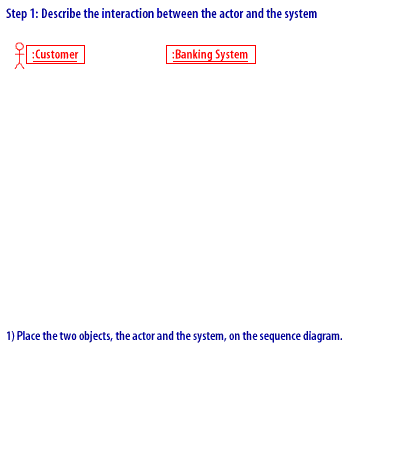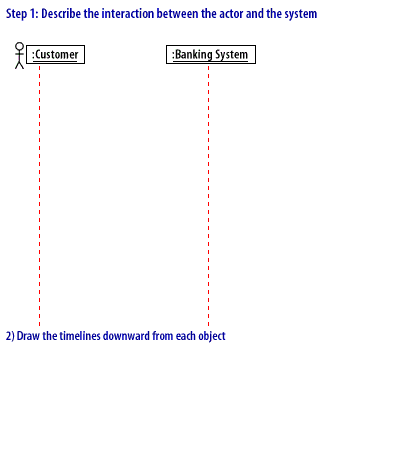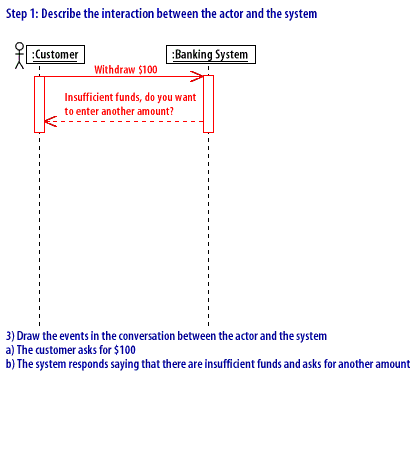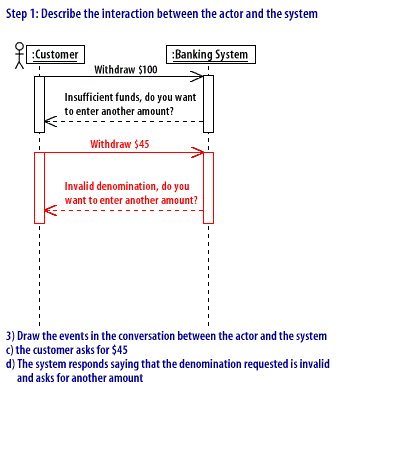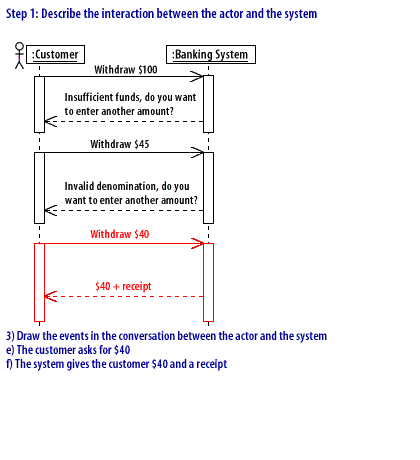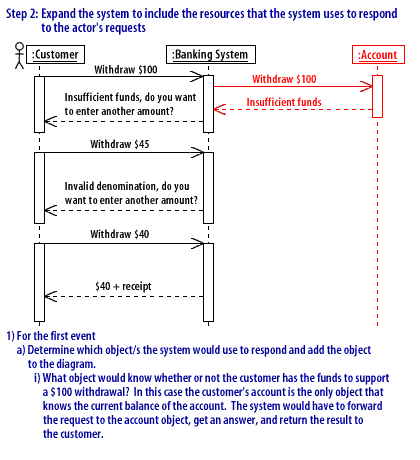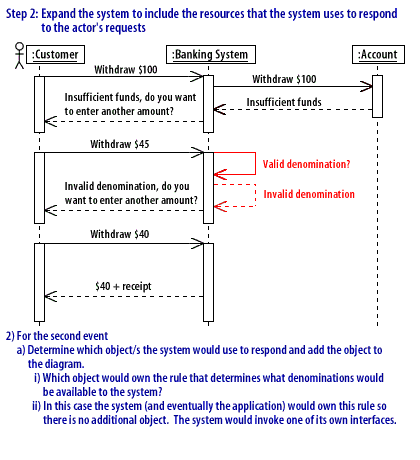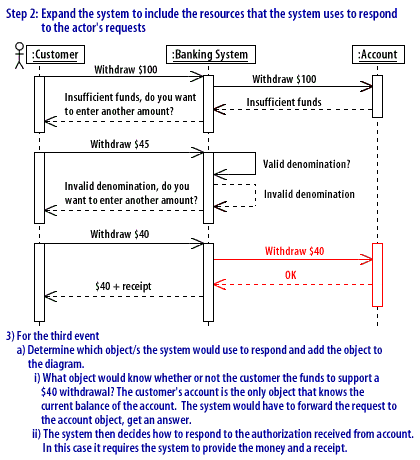Building Sequence Diagram

### Caution: keep it simple

Most use cases include decision logic. The event flows resulting from multiple decisions can become very complex. Technically, the Unified Modeling Language (UML) sequence diagram supports branching. But the resulting diagram is very difficult to read, and no tools currently support this option. For these reasons, I strongly recommend that you limit a sequence diagram to one scenario.
A scenario is a single logic path through a use case. In the following lessons, you will learn how to use scenarios to build your sequence diagrams.

### Applying Sequence Diagram - Quiz

Click the Quiz link below to take a short multiple-choice quiz on sequence diagram notation.
Applying Sequence Diagram - Quiz